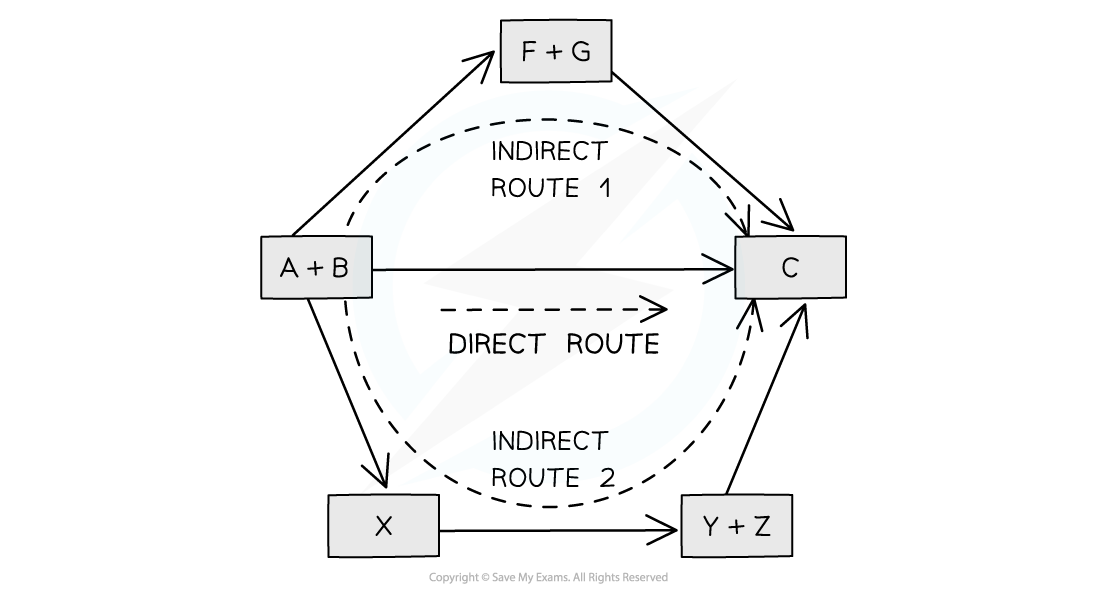# IB DP Chemistry: SL复习笔记5.2.1 Hess's Law

### Hess's Law

• In 1840, the Russian chemist Germain Hess formulated a law which went on to be known as Hess’s Law
• This went on to form the basis of one of the laws of thermodynamics. The first law of thermodynamics relates to the Law of Conservation of Energy
• It is sometimes expressed in the following form:

Energy cannot be created or destroyed, it can only change form

• This means that in a closed system, the total amount of energy present is always constant
• Hess’s law can be used to calculate the standard enthalpy change of a reaction from known standard enthalpy changes
• Hess’s Law states that:

"The total enthalpy change in a chemical reaction is independent of the route by which the chemical reaction takes place as long as the initial and final conditions are the same."

• This means that whether the reaction takes place in one or two steps, the total enthalpy change of the reaction will still be the sameThe diagram above illustrates Hess’ Law: the enthalpy change of the direct route, going from reactants (A+B) to product (C) is equal to the enthalpy change of the indirect routes

• Hess’ Law is used to calculate enthalpy changes which can’t be found experimentally using calorimetry, eg:

3C (s) + 4H(g) → C3H8(g)

• [popover id="dGQl3oDiqUIQrR1A" label="ΔHf"] , ΔHf (propane) can’t be found experimentally as hydrogen and carbon don’t react under standard conditions

#### Calculating ΔHr from ΔHf using Hess’s Law energy cycles

• You can see the relationships on the following diagram:The enthalpy change from elements to products (direct route) is equal to the enthalpy change of elements forming reactants and then products (indirect route)

• The products can be directly formed from the elements = ΔH2

OR

• The products can be indirectly formed from the elements = ΔH1 + ΔHr
• Equation

ΔH2 = ΔH1 + ΔHr

Therefore for energy to be conserved,

ΔH= ΔH2 – ΔH1

#### Exam Tip

You do not need to learn Hess's Law word for word as it is not a syllabus requirement, but you do need to understand the principle as it provides the foundation for all the problem solving in Chemical Energetics